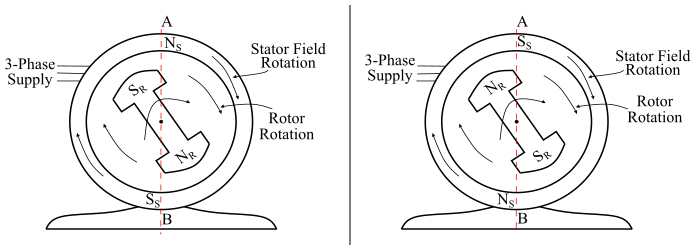# AC Motor Types: Synchronous Motor & Induction Motor

An AC motor is an electromechanical device which converts electrical energy input into mechanical energy. The AC motors are mainly classified into two types viz.

• Asynchronous or Induction Motor
• Synchronous Motor

## Asynchronous or Induction Motor

As the name implies, the asynchronous motors are the ones whose speed is not equal to synchronous speed, i.e. these motors run at a speed slightly less than the synchronous speed. The induction motors are the types of asynchronous motors.The induction motor consists of a stator and a rotor. The stator carries a 3-phase winding while the rotor carries a short circuited winding. When the stator winding is energised from a 3-phase supply, a rotating magnetic field is set up which rotates around the stator at synchronous speed. This rotating magnetic field passes through the air-gap and cuts the rotor conductors, which are stationary. Therefore, due to relative speed between the rotating magnetic field and the stationary rotor conductors, an EMF is induced in the rotor conductors. As the rotor circuit is short circuited, currents start flowing in the rotor conductors.

The current carrying rotor conductor are placed in the magnetic field produced by the stator winding. As a result of this, a torque develops on the rotor conductors and the sum of the torque on all the rotor conductors, rotates the rotor in the same direction as the rotating magnetic field but at a speed slightly less than the synchronous speed, because if the rotor attains the synchronous speed there is no relative motion between the rotor and rotating magnetic field and hence no electromagnetic torque on the rotor conductors. This is how, an asynchronous or induction motor works.

## Synchronous Motors

Those AC motors whose rotor rotates on the synchronous speed of the rotating magnetic field are known as synchronous AC motors. The synchronous motors are the constant speed (equal to synchronous speed) motors at all loads provided that the load on the motor does not exceed the limiting value. If the load on the shaft of a synchronous motor exceeds the limiting value, then the motor comes to rest and the average torque developed by it is zero.

The synchronous motor has a stator and a rotor. The stator consists of a 3-phase armature winding and receives power from a balanced 3-phase supply while the rotor has a set of salient poles excited by direct current to produce alternate N and S poles. In case of a synchronous motor, the stator is wound for the same number of poles as the rotor poles.

## Operation of Synchronous Motors

Consider a synchronous motor having two rotor poles NR and SR, then the stator will also be wound for two poles NS and SS. A DC voltage is applied to the rotor winding and a 3-phase supply is connected to the stator winding. The stator winding produces a rotating magnetic field which revolves around the stator at synchronous speed. Thus, we have a situation in which there exists a pair of stationary rotor poles (NR-SR) and a pair of revolving armature or stator poles (NS-SS).

Now, let at any instant, the stator poles are at positions A and B (see the figure). It is clear that poles NS and NR repel each other and so do the poles SS and SR. Thus, the rotor tends to move in anti-clockwise direction.After a period of half cycle, the polarities of stator poles are reversed but the polarities of the rotor poles remain the same (see the figure). Now, SS and NR attract each other and so do the NS and SR. Therefore, the rotor tends to move in clockwise direction.From the above discussion, it is clear that the stator poles change their polarities rapidly and they tend to pull the rotor first in one direction and then after the period of half cycle in the other direction. Because of high inertia of rotor, the motor fails to start. Hence, a synchronous motor cannot start by itself.Now, if the rotor poles are rotated by some external means at such a speed that they interchange their positions along with the stator poles and being magnetically locked with stator poles. Then the rotor will experience a continuous unidirectional torque. Hence, the synchronous motor now, runs at the speed of revolving magnetic field or synchronous speed.